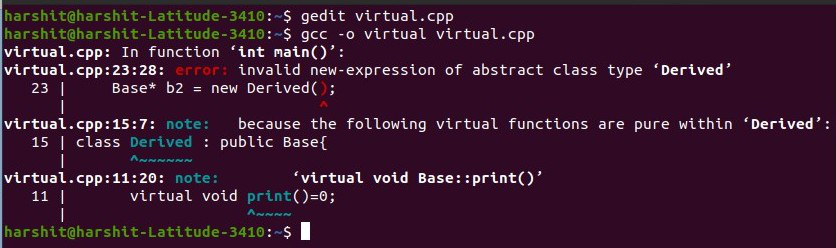Open in App
Not now

# Behavior of virtual function in the derived class from the base class and abstract class

• Difficulty Level : Hard
• Last Updated : 06 Apr, 2021

In this article, we will discuss the behavior of Virtual Function in the derived class and derived class from the Abstract Base Class in C++.

Consider the following program:

## C++

 `// C++ program to illustrate the concept``// of Virtual Function`` ` `#include ``using` `namespace` `std;`` ` `// Base Class``class` `Base {``public``:``    ``// Virtual Function``    ``virtual` `void` `print()``    ``{``        ``cout << ``"Inside Base"` `<< endl;``    ``}``};`` ` `// Derived Class``class` `Derived : ``public` `Base {``};`` ` `// Driver Code``int` `main()``{`` ` `    ``// Object of the derived class``    ``Base* b2 = ``new` `Derived();`` ` `    ``// Function Call to Base Class``    ``b2->print();`` ` `    ``return` `0;``}`

Output:

```Inside Base
```

Explanation: When a pointer of the Base Class is declared pointing to an object of the Derived Class, then only declare the function as virtual in the base when it is needed to override it in the derived class. If the function is declared as a virtual in the Base Class, then it is not recommended to override it in the derived class, it will still call the virtual function and execute it.

The virtual keyword only works when going for runtime polymorphism and overriding the function of the base class in the derived class. If a virtual keyword is used, and it is not recommended to override that function in the derived class, then the virtual keyword is of no use. This property doesn’t hold true for the Abstract Base class because there is no function body in the Base class as well. Below is the program to illustrate the same:

Program 1:

## C++

 `// C++ program to illustrate the concept``// of virtual function`` ` `#include ``using` `namespace` `std;`` ` `// Base Class``class` `Base {``public``:``    ``// Virtual Function``    ``virtual` `void` `print() = 0;``};`` ` `// Derived Class``class` `Derived : ``public` `Base {`` ` `    ``// Overriding of virtual function``    ``void` `print()``    ``{``        ``cout << ``"Inside Derived"`` ``<< endl;``    ``}``};`` ` `// Driver Code``int` `main()``{``    ``// Object of the derived class``    ``Base* b2 = ``new` `Derived();`` ` `    ``// Function Call to Base Class``    ``b2->print();`` ` `    ``return` `0;``}`

Output:

```Inside Derived
```

Program 2:

## C++

 `// C++ program to illustrate the concept``// of virtual function`` ` `#include ``using` `namespace` `std;`` ` `// Base Class``class` `Base {``public``:``    ``// Virtual Function``    ``virtual` `void` `print() = 0;``};`` ` `// Derived Class``class` `Derived : ``public` `Base {``};`` ` `// Driver Code``int` `main()``{`` ` `    ``// Object of the derived class``    ``Base* b2 = ``new` `Derived();`` ` `    ``// Function Call to Base Class``    ``b2->print();`` ` `    ``return` `0;``}`

Output:Explanation: If the above two programs are compared, when the function is overridden of the abstract class, then the virtual keyword is working the way it has been intended to work. But in the above program, the function wasn’t overridden inside the Base Class, and also the function was not defined in the Base Class, result in the Compilation Error.

My Personal Notes arrow_drop_up